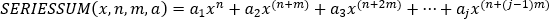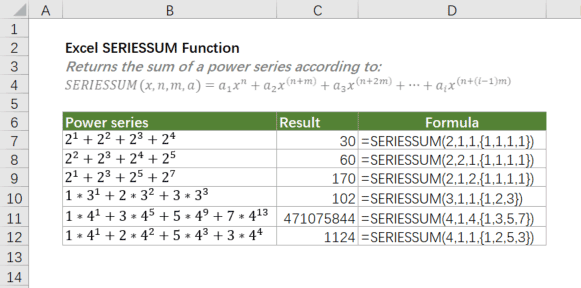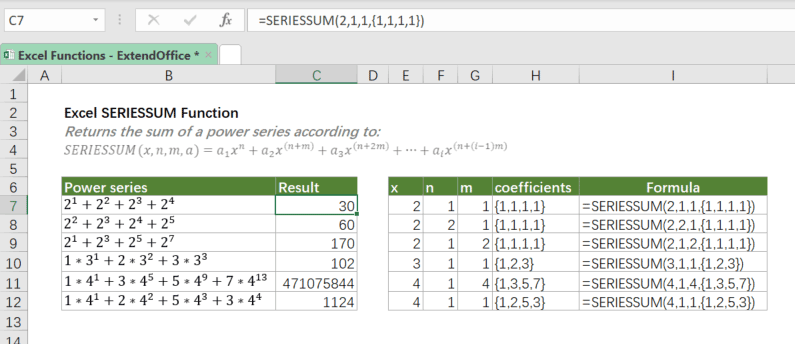Note: The other languages of the website are Google-translated. Back to English

## Excel SERIESSUM Function

The SERIESSUM function returns the sum of a power series, based on the following formula:Where i is the number of entries of a.#### Syntax

=SERIESSUM(x, n, m, coefficients)

#### Arguments

• x (required): The input value to the power series.
• n (required): The initial power to which x is raised in the power series.
• m (required): The step by which n is increased for each term in the series.
• coefficients (required): A set of coefficients ({a1,a2,…,ai}) that multiply each successive power of x.

#### Return Value

The SERIESSUM function returns a numeric value.

#### Function Notes

• The number of values in coefficients determines the number of terms in the power series. For example, if there are four values in coefficients, then there will be four terms in the power series.
• SERIESSUM returns the #VALUE! error value if any argument is non-numeric.

#### Example

As shown in the table below, we have several power series expansions. To get their results with SERIESSUM, we should first figure out what x, n, m and coefficients are for each expansion according to the formula the SERIESSUM uses, and then add them to the SERIESSUM function in sequence as below formulas shown.#### Related functions

Excel SUM Function

The SUM function adds all values you specified. The values can be numbers, cell references, ranges, arrays or a mix of all four.

Excel SUMSQ Function

The SUMSQ function returns the sum of squares of supplied values.

Excel SUMIF Function

In Excel, the SUMIF function can help to sum cells based on one criterion, this article will explain this function's syntax and introduce some examples for using the SUMIF function.

### The Best Office Productivity Tools

#### Kutools for Excel - Helps You To Stand Out From Crowd

Would you like to complete your daily work quickly and perfectly? Kutools for Excel brings 300 powerful advanced features (Combine workbooks, sum by color, split cell contents, convert date, and so on...) and save 80% time for you.

• Designed for 1500 work scenarios, helps you solve 80% Excel problems.
• Reduce thousands of keyboard and mouse clicks every day, relieve your tired eyes and hands.
• Become an Excel expert in 3 minutes. No longer need to remember any painful formulas and VBA codes.
• 30-day unlimited free trial. 60-day money back guarantee. Free upgrade and support for 2 years.#### Office Tab - Enable Tabbed Reading and Editing in Microsoft Office (include Excel)

• One second to switch between dozens of open documents!
• Reduce hundreds of mouse clicks for you every day, say goodbye to mouse hand.
• Increases your productivity by 50% when viewing and editing multiple documents.
• Brings Efficient Tabs to Office (include Excel), Just Like Chrome, Firefox, And New Internet Explorer.